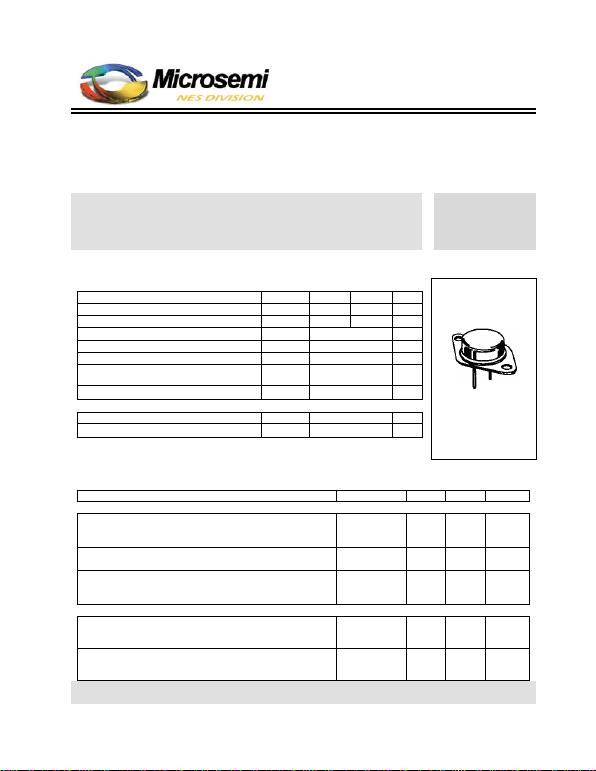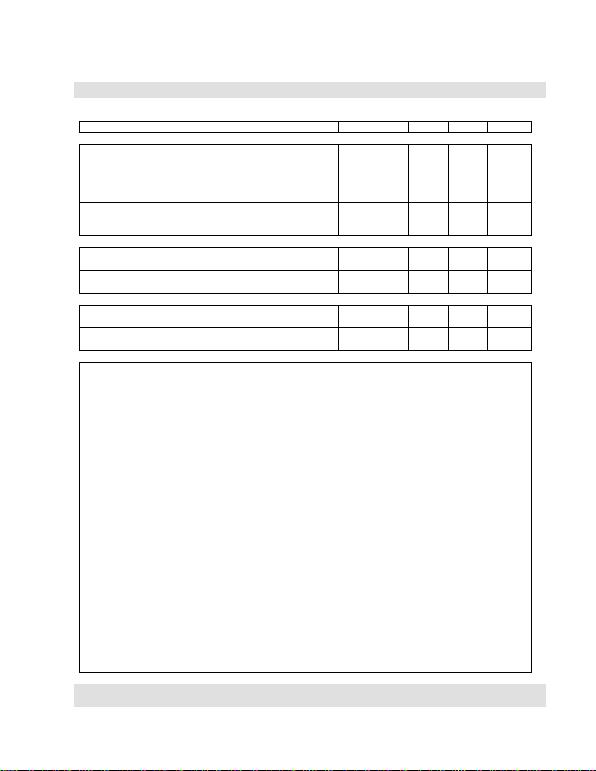TECHNICAL DATA
NPN HIGH POWER SILICON TRANSISTOR
Qualified per MIL-PRF-19500/371
Devices
Qualified Level
2N3902 2N5157
JAN
JANTX
MAXIMUM RATINGS
Ratings Symbol 2N3902 2N5157 Unit
Collector-Emitter Voltage VCEO 400 500 Vdc
Emitter-Base Voltage VEBO 5.0 6.0 Vdc
Collector-Base Voltage VCBO 700 Vdc
Total Power Dissipation @ TA = +250C (1)
@ TC = +750C (2) PT 5.0
100 W
W
Operating & Storage Temperature Range Tj, Tstg -65 to +200 0C
THERMAL CHARACTERISTICS
Characteristics Symbol Max. Unit
Thermal Resistance, Junction-to-Case RθJC 1.25 0C/W
1) Derate linearly 29 mW/0C for TA > +250C
2) Derate linearly 0.8 mW/0C for TC > +750C
TO-3 (TO-204AA)*
*See Appendix A for Package
Outline
ELECTRICAL CHARACTERISTICS
Characteristics Symbol Min. Max. Unit
OFF CHARACTERISTICS
Collector-Emitter Cutoff Current
VCE = 325 Vdc 2N3902
VCE = 400 Vdc 2N5157 ICEO 250
Collector-Emitter Cutoff Current
VBE = 1.5 Vdc; VCE = 700 Vdc ICEX 500 µAdc
Emitter-Base Cutoff Current
VEB = 5.0 Vdc 2N3902
VEB = 6.0 Vdc 2N5157 IEBO 200
ON CHARACTERISTICS(3)
Base-Emitter Saturation Voltage
1.5
2.0 Vdc
Collector-Emitter Saturation Voltage
2.5 Vdc
6 Lake Street, Lawrence, MA 01841
1-800-446-1158 / (978) 794-1666 / Fax: (978) 689-0803
120101
Page 1 of 22N3902, 2N5157 JAN SERIES
ELECTRICAL CHARACTERISTICS (con’t)
Characteristics Symbol Min. Max. Unit
ON CHARACTERISTICS(3) (con’t)
Forward-Current Transfer Ratio
IC = 0.5 Adc; VCE = 5.0 Vdc
IC = 1.0 Adc; VCE = 5.0 Vdc
IC = 2.5 Adc; VCE = 5.0 Vdc
IC = 3.5 Adc; VCE = 5.0 Vdc
hFE
25
30
10
5
90
Collector-Emitter Sustaining Voltage
2N5157 VCEO(sus) 325
400 Vdc
DYNAMIC CHARACTERISTICS
Small-Signal Short-Circuit Forward Current Transfer Ratio
IC = 0.2 Adc; VCE = 10 Vdc, f = 1 MHz hfe 2.5 25
Output Capacitance
VCB = 10 Vdc; IE = 0, 100 kHz f 1.0 MHz Cobo 250 pF
SWITCHING CHARACTERISTICS
Turn-On Time
VCC = 125 Vdc; IC = 1.0 Adc; IB1= 0.1 Adc ton 0.8 µs
Turn-Off Time
VCC = 125 Vdc; IC = 1.0 Adc; IB1 = 0.1 Adc; -IB2 = 0.50 Adc toff 1.7 µs
SAFE OPERATING AREA
DC Tests (continuous)
TC = +250C; t 1.0 s (See Figure 3 of MIL-PRF-19500/371)
Test 1
VCE = 28.6 Vdc, IC = 3.5 Adc
Test 2
VCE = 70 Vdc, IC = 1.43 Adc
Test 3
VCE = 325 Vdc, IC = 55 mAdc 2N3902
VCE = 400 Vdc, IC = 35 mAdc 2N5157
Switching Tests
TC = 250C; duty cycle 10%; RS = 0.1 (See Figure 4 of MIL-PRF-19500/371)
Test 1
tP = approximately 3 ms (vary to obtain IC); RBB1 = 20 ; VBB1 = 10 Vdc; RBB2 = 3 k;
VBB2 = 1.5 Vdc; VCC = 50 Vdc; IC = 3.5 Adc; L = 60 mH; R = 3 ; RL 14.
Test 2
tP = approximately 3 ms (vary to obtain IC); RBB1 = 100 ; VBB1 = 10 Vdc; RBB2 = 3 k;
VBB2 = 1.5 Vdc; IC = 0.6 Adc VCC = 50 Vdc; L = 200 mH; R = 8 ; RL 83.
Switching Tests